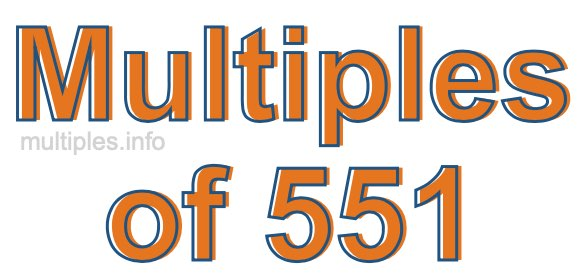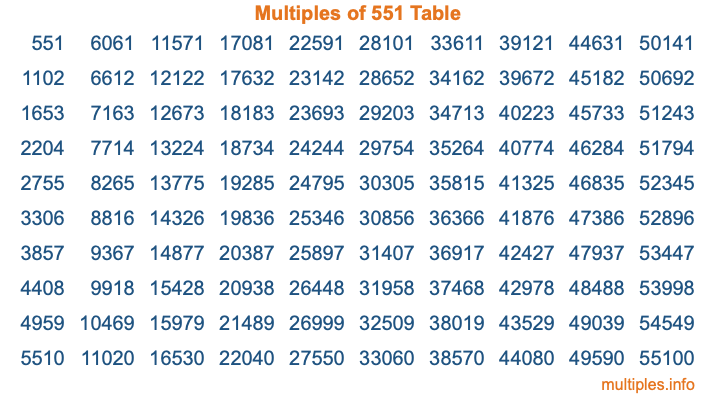Multiples of 551Welcome to the Multiples of 551 page. Here we will first teach you everything you will ever need to know about the multiples of 551, and then give you a study guide summary of everything we taught you to make sure you remember it all. Use this page to look up facts and learn information about the multiples of 551. This page will make you a multiples of five hundred fifty-one expert!

Definition of Multiples of 551
Multiples of 551 are all the numbers that when divided by 551 equal an integer. Each of the multiples of 551 are called a multiple. A multiple of 551 is created by multiplying 551 by an integer.

Therefore, to create a list of multiples of 551, you start with 1 multiplied by 551, then 2 multiplied by 551, then 3 multiplied by 551, and so on for as long as you want. Thus, the list of the first five multiples of 551 is 551, 1102, 1653, 2204, and 2755. To see a larger list of multiples of 551, see the printable image of Multiples of 551 further down on this page. We also have a category where you can choose any nth multiple of 551.

Multiples of 551 Checker
The Multiples of 551 Checker below checks to see if any number of your choice is a multiple of 551. In other words, it checks to see if there is any number (integer) that when multiplied by 551 will equal your number. To do that, we divide your number by 551. If the the quotient is an integer, then your number is a multiple of 551.

Is  a multiple of 551?

Least Common Multiple of 551 and ...
A Least Common Multiple (LCM) is the lowest multiple that two or more numbers have in common. This is also called the smallest common multiple or lowest common multiple and is useful to know when you are adding our subtracting fractions. Enter one or more numbers below (551 is already entered) to find the LCM.

Check out our LCM Calculator if you need more details about the Least Common Multiple or if you need the LCM for different numbers for adding and subtraction fractions.

nth Multiple of 551
As we stated above, 551 is the first multiple of 551, 1102 is the second multiple of 551, 1653 is the third multiple of 551, and so on. Enter a number below to find the nth multiple of 551.

th multiple of 551

Multiples of 551 vs Factors of 551
551 is a multiple of 551 and a factor of 551, but that is where the similarities end. All postive multiples of 551 are 551 or greater than 551. All positive factors of 551 are 551 or less than 551.

Below is the beginning list of multiples of 551 and the factors of 551 so you can compare:

Multiples of 551: 551, 1102, 1653, 2204, 2755, etc.

Factors of 551: 1, 19, 29, 551

As you can see, the multiples of 551 are all the numbers that you can divide by 551 to get a whole number. The factors of 551, on the other hand, are all the whole numbers that you can multiply by another whole number to get 551.

It's also interesting to note that if a number (x) is a factor of 551, then 551 will also be a multiple of that number (x).

Multiples of 551 vs Divisors of 551
The divisors of 551 are all the integers that 551 can be divided by evenly. Below is a list of the divisors of 551.

Divisors of 551: 1, 19, 29, 551

The interesting thing to note here is that if you take any multiple of 551 and divide it by a divisor of 551, you will see that the quotient is an integer.

Multiples of 551 Table
Below is an image of the first 100 multiples of 551 in a table. The table is in chronological order, column by column. The first column has the first ten multiples of 551, the second column has the next ten multiples of 551, and so on.The Multiples of 551 Table is also referred to as the 551 Times Table or Times Table of 551. You are welcome to print out our table for your studies.

Negative Multiples of 551
Although not often discussed or needed in math, it is worth mentioning that you can make a list of negative multiples of 551 by multiplying 551 by -1, then by -2, then by -3, and so on, to get the following list of negative multiples of 551:

-551, -1102, -1653, -2204, -2755, etc.

Multiples of 551 Summary
Below is a summary of important Multiples of 551 facts that we have discussed on this page. To retain the knowledge on this page, we recommend that you read through the summary and explain to yourself or a study partner why they hold true.

There are an infinite number of multiples of 551.

A multiple of 551 divided by 551 will equal a whole number.

551 divided by a factor of 551 equals a divisor of 551.

The nth multiple of 551 is n times 551.

The largest factor of 551 is equal to the first positive multiple of 551.

551 is a multiple of every factor of 551.

551 is a multiple of 551.

A multiple of 551 divided by a divisor of 551 equals an integer.

551 divided by a divisor of 551 equals a factor of 551.

Any integer times 551 will equal a multiple of 551.

Multiples of a Number
Here you can get the multiples of another number, all with the same attention to detail as we did for multiples of 551 on this page.

Multiples of
Multiples of 552
Did you find our page about multiples of five hundred fifty-one educational? Do you want more knowledge? Check out the multiples of the next number on our list!